# 5 Generations of CPU Heat Issues Resolved

What happens when below dewpoint cooling is combined with two different water pressures? We started at 85°C then increased the temperature 50% for four successive generations: first to fifth generation chips are respectively 85°C, 127.5°C, 191.25°C, 286.88°C, and 430.31°C. The optimal working temperature of the chip is 70°C.

When using circulating fluid at various temperatures, one must resolve the issues of condensation both on the fluid containment unit and on and around the CPU. Our protection patents resolve condensation by wicking the moisture away from the CPU and motherboard to be evaporated by the heat of the motherboard itself. The second part of the patents is a simple but elegant water barrier protection system, which keeps the electronic components safe from moisture.

We focused on two variables only: flow rate and temperature. Every data center will have its own unique set-up in terms of size, number of server racks, layout, CPU brand, etc.

##### Problem by Advanced Computer Chilling By P Kaushik, PhD September 27, 2022

Based on the requirements given by Dr. H Christian Gunderson (CEO of Advanced Computer Chilling), we need to replace air cooling with water cooling. This has to be achieved by using the simplest model. The following steps are the way in which the goal is achieved.

Let us consider a microprocessor chip to be cooled as shown in Fig. 1. The chip is cooled using fan(s) blown air with air velocity as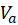at temperature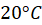. The length of the chip in the direction of air flow is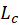and area of top of chip in contact with blowing air is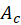. Depending on the generation of the processor the temperature at which the chip operates is given by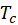. The suffix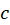is used for chip.Fig. 1. Cooling of microprocessor chip by air blown over it.

Given data states thatfor first to fifth generation chips are respectively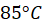,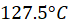,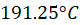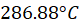and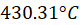. The optimal working temperature of the chip is 70°C. We assume that the air-cooled chips are operating at the above given temperatures for each generation.

Typically for typical range of CPU fan speeds, the flow of air over chip will be laminar and therefore, the average Nusselt number (average dimensionless heat transfer parameter) can be expressed in the well know correlation as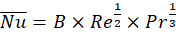, where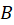is constant with value 0.664. The flow Reynolds number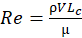and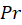is the Prandtl number, which for air is about 0.71. Here,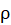is the density of the fluid,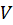is the velocity of flow over the microprocessor in m/s and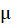is the dynamic viscosity of the liquid flowing over the chip. The heat transfer coefficient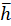may be determined from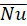using,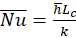where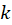is the thermal conductivity of the fluid. After determiningfrom the above relation for air, we can determine the heat carried in watts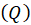away by air at steady state operation for each generation of chips, given by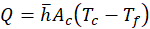Eq. 1

where,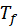is the fluid temperature. Using the relation forin terms of average Nusselt number, considering the flow to be similar to a laminar flat plate boundary layer , we get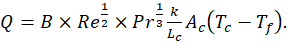Eq. 2

We may further expand the relation using the expression for Re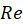stated above, to get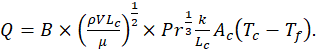Eq. 3

Using the suffix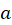to indicate properties with respect to air or air flow, we get, the rate of heat taken away by air under steady state operation as: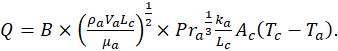Eq. 4

The rate of heat taken away by air at steady state operation is the same as the rate of heat produced by the microprocessor chip. This heat is to be removed using chilled water as suggested by Dr. H Christian Gunderson (CEO of Advanced Computer Chilling). Therefore, we replace the above formula in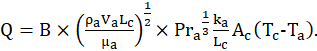Eq. 4 with suffix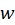to indicate the properties of water or water flow, to get,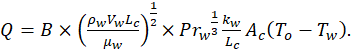Eq. 5

Note that in the above equation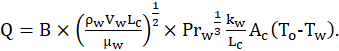Eq. 5, the chip temperaturehas been replaced with operating temperature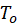, since we want the chip to operate the chosen optimal temperature of 70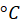. We need to determine the water temperature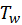and we assume the the chip was cooled using air at 20in case of Eq. 4. We equate Eqs. 4 and 5 to get an equation for the temperature of water needed, given by: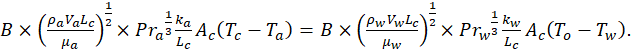Eq. 6

Simplifying Eq. 6, we get,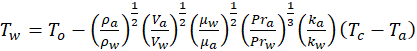Eq. 7

We take the properties of both water and air at average operating temperature. We take the density of air as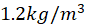and water as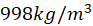. The viscosity of air as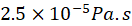and of water as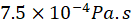. The Prandtl numbers for water and air are respetively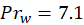and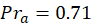. The thermal conductivity of air is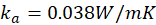and water is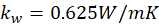. Taking air temperature for regular cooling as, optimal operating temperature as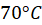and substituting the values, we get,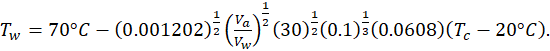Eq. 8

On further simplification,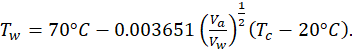Eq. 9

Typically, the flow speed of water required will be much lower than air speed as the heat capacity of water is orders of magnitude higher. Therefore, we plot the water temperatureneeded to keep the chip at optimaltemperature as a function of the ratio of air flow speed to the water flow speed. We take the ratio of air flow speed to be 100 times the water flow speed and vary it up to 10000 times the water flow speed and plot it in Fig. 2.Fig. 2. The inlet temperature of waterinneeded to keep chip of each generation at optimalas a function of the ratio of air flow speed to the water flow speed. The horizontal axis has been taken in the log scale.

We note from Fig. 2 that the water temperature at inlet to the chip decreases quite slowly with increasing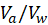ratio for lower values of(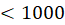). Therefore, the operation of the cooling system should prefer values of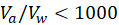. Further, we see that for higher generations of the chips the water inlet temperature reduces quite drastically with increase inratio. In order to reduce the chilling requirement of water, the flow rate (velocity) of the water should be increased to keep the chilling cost low. However, the pumping cost will certainly increase when the flow rate of water is increased. The operating cost should consider both these opposing parameters.

To make the choice of water inlet temperature, even simpler, the speed at which fan blows air over the chip is taken at an average speed of approximately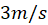. Typically, to pump water, more energy is required and therefore, we can reduce pumping power by reducing the speed of flow to about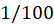th to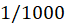th the average speed of air, which gives the average water flow speed over the chip as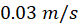to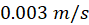. Considering, these values, we can tabulate the choice of range ofin the following table:For any further questions or queries, you may contact me at kaushik.engg@gmail.com or at https://www.linkedin.com/in/p-kaushik-phd-1b04ab145/

Conclusion:

We resolved CPU heat issues for five generations using two variables: temperature and flow rate. Using our patented condensation and water barrier protection systems will allow for maximum flexibility in setting both variables – going below dewpoint becomes a viable option.

We invite you to look at P Kaushik, PhD's calculations and test our conclusions according to your company’s set-up and needs.